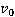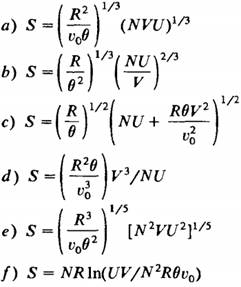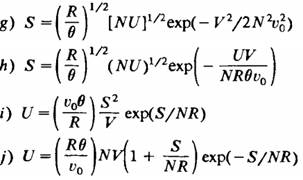### Create an Account

Home / Questions / For each of the five physically acceptable fundamental equations in problem 110 1 find U a...

# For each of the five physically acceptable fundamental equations in problem 110 1 find U as a function of S V and N Problem 110 1 The following ten equations are purported to be fundamental

For each of the five physically acceptable fundamental equations in problem 1.10-1 find U as a function of S, V, and N.

Problem 1.10-1

The following ten equations are purported to be fundamental equations of various thermodynamic systems. However, five are inconsistent with one or more of postulates II, III, and IV and consequently are not physically acceptable. In each case qualitatively sketch the fundamental relationship between S and U (with N and V constant). Find the five equations that are not physically permissible and indicate the postulates violated by each. The quantities, θ, and R are positive constants, and in all cases in which fractional exponents appear only the real positive root is to be taken.Jun 09 2020 View more View LessSubscribe To Get Solution